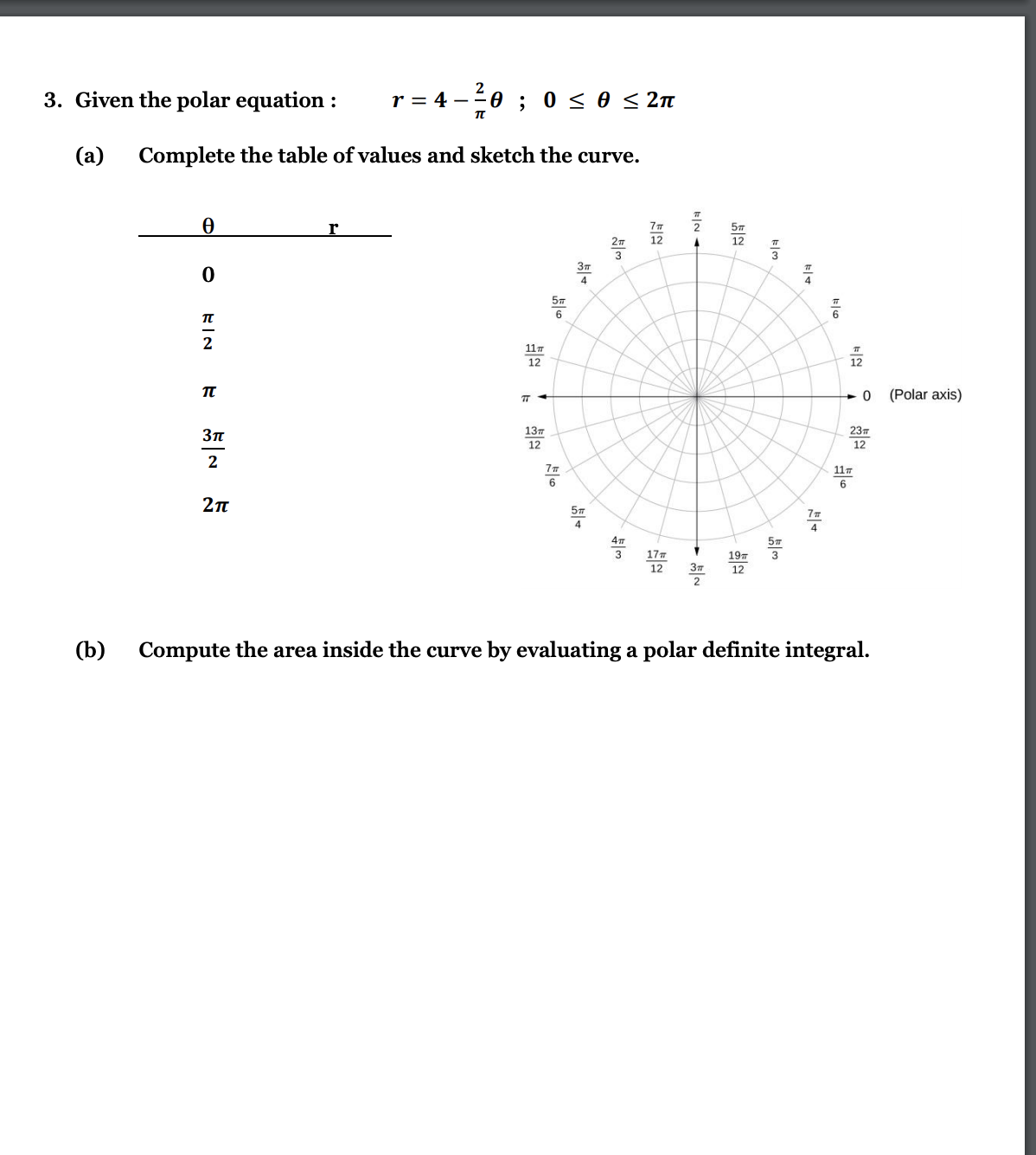YY

Yaboiimarco Y.

Calculus 2 / BC

3 days, 2 hours ago

Step by stepStep by step

### Discussion

You must be signed in to discuss.

### Video Transcript

All right, so we're problem 23. We have to sketch a graph of the given region and evaluates the integral. So first, a little sketch the graph toe, figure out our boundaries. So we've got our axes and we'll start with the fate of boundaries for us. So if they the issue how much here rotates counterclockwise from the positive x axis. So you started zero, which is the positives of X axis. And then you end that two pi, which means you go a full circle and end up at the positive X axis. So now for our founders, well, R is the distance from the Oregon. So we started a zero, which is the origin, and then we end up for which is four years away from the Oregon. So our region of integration is gonna look like this. Ah, circle of radios. For and with that we can go on and set up our and double fuller into girls. So basically we have are two into girls and then we have our into grand all multiplied by r. And then we have a DRD data. So let's figure out our boundaries. So for our is from 0 to 4 and we see that DRS stick on the inside. So in the inside, into broken that go from 0 to 4 and then for 1/3 of boundaries is gonna go from 0 to 5. Now, before we continue, we have to converts of this X and y into polar coordinates. Well, one thing that's a uni remembers that are SKorea's you goto Xcor close ally script and you can simply derive this from the Pythagorean zero. So if you have a point of coordinates x and Y, then the distance from the point Teoh, the origin is gonna follow this formula. And with we're just gonna replace this ex current and first wife screamed with our scoring. So in the end are integral is gonna be 0 to 2 pi 0 to 4. Our score times are isn t r cubed. Remember, you have, like another you are here and then it's gonna give the authority say that And with that, we can go on with evaluating. Now I'm going to use a few REM which is going to go us follows eventually this If your own states that if the boundaries of all the integral are constant. Then you're allowed to, like, split this double, integral into two, separate into gross and then multiply them together. So if you're going to see we split up off like the integral with the d r from the integral with a d state s O are integral with the DRS gonna have the are accused distance you have to, like, bring along is the corresponding variables And yeah, we just like you, Violet. Every evaluates them from here. So things grow off our cue in this game, 1/4 are to the fourth from for 20 And the way we could just imagine that there's like of one here. So the integral of one's just gonna be with the respective day does that's going to faint and is going to be from two clients. Zero 1/4 times, four to the fourth, which is 250 snakes minus 1/4 time, zero to the fourth. Well, it's just gonna be zero. And on the right side, we have to climb Linus zero, which is just gonna be to pine. And we have 256 divided my floor, which is gonna be a 64 and we have to apply on the right, and then we multiply them together. So in the end, we're gonna have 128 5 and this will be our answer. So remember, if you want to, like, split off, like unless the into girl or when you have, like, into girls inside of another integral, you can only do it like it If all of the boundaries are constant. If any of the boundaries that is a function, then you're gonna have to integrate from the inside out. But yeah, this is basically it's##### Top Calculus 2 / BC Educators##### Catherine R.

Missouri State University##### Samuel H.

University of Nottingham